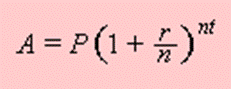Welcome, fellow mathematicians, to my very first post about mathematics. Here’s where I’ll delve into my quest of understanding math mysteries, and.. formulas.  I don’t claim to know much about math, in fact, I know very little of it, but I’m always interested in the why of things. In each of the posts on this page, I’ll be trying to understand mathematics concepts. And if I’m in an impasse, I’ll be relying on you for help!P = principal amount (the initial amount you borrow or deposit)

r  = annual rate of interest (as a decimal)

t  = number of years the amount is deposited or borrowed for.

A = amount of money accumulated after n years, including interest.

n  =  number of times the interest is compounded per year

I’m trying to proof that this formula works. (I know it does) But how do I do that? Tap tap, click click…